# ggplot2: Line Graphs

### Introduction

This is the 8th post in the series Elegant Data Visualization with ggplot2. In the previous post, we learnt to build scatter plots. In this post, we will learn to:

• build
• simple line chart
• grouped line chart
• map aesthetics to variables
• modify line
• color
• type
• size

### Libraries, Code & Data

We will use the following libraries in this post:

All the data sets used in this post can be found here and code can be downloaded from here.

#### Case Study

We will use a data set related to GDP growth rate. You can download it from here. It contains GDP (Gross Domestic Product) growth data for the BRICS (Brazil, Russia, India, China, South Africa) for the years 2000 to 2005.

#### Data

``gdp <- readr::read_csv('https://raw.githubusercontent.com/rsquaredacademy/datasets/master/gdp.csv')``
``## Warning: Missing column names filled in: 'X1' ``
``gdp``
``````## # A tibble: 6 x 6
##      X1     X year       growth india china
##   <dbl> <dbl> <date>      <dbl> <dbl> <dbl>
## 1     1     1 2000-01-01      6     5     8
## 2     2     2 2001-01-01      9     9     5
## 3     3     3 2002-01-01      8     8     6
## 4     4     4 2003-01-01      9     8     8
## 5     5     5 2004-01-01      9     5     9
## 6     6     6 2005-01-01      8     7     8``````

#### Line Chart

To create a line chart, use `geom_line()`. In the below example, we examine the GDP growth rate trend of India for the years 2000 to 2005.

``````ggplot(gdp, aes(year, india)) +
geom_line()``````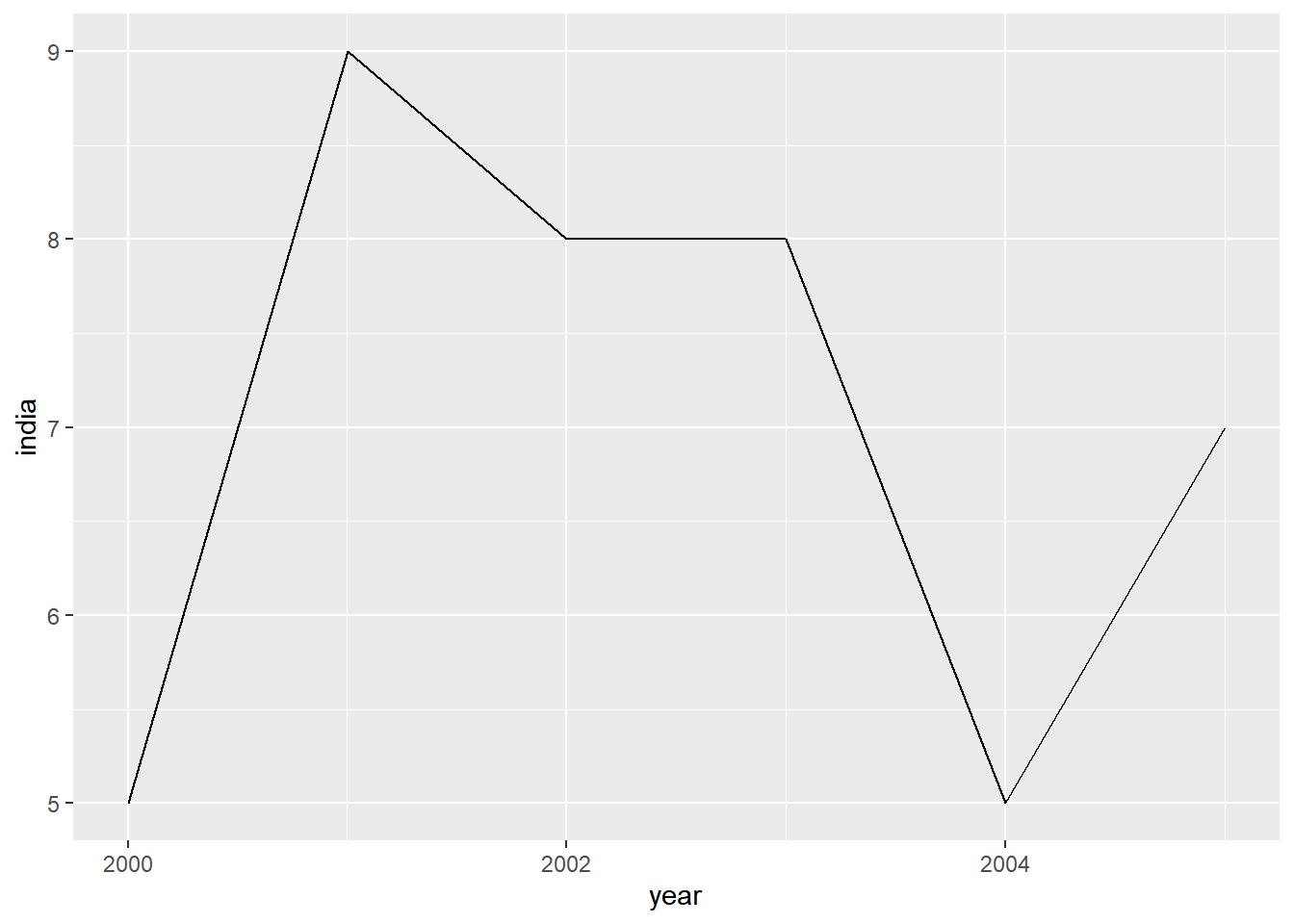#### Line Color

To modify the color of the line, use the `color` argument and supply it a valid color name. In the below example, we modify the color of the line to `'blue'`. Remember that the `color` argument should be outside `aes()`.

``````ggplot(gdp, aes(year, india)) +
geom_line(color = 'blue')``````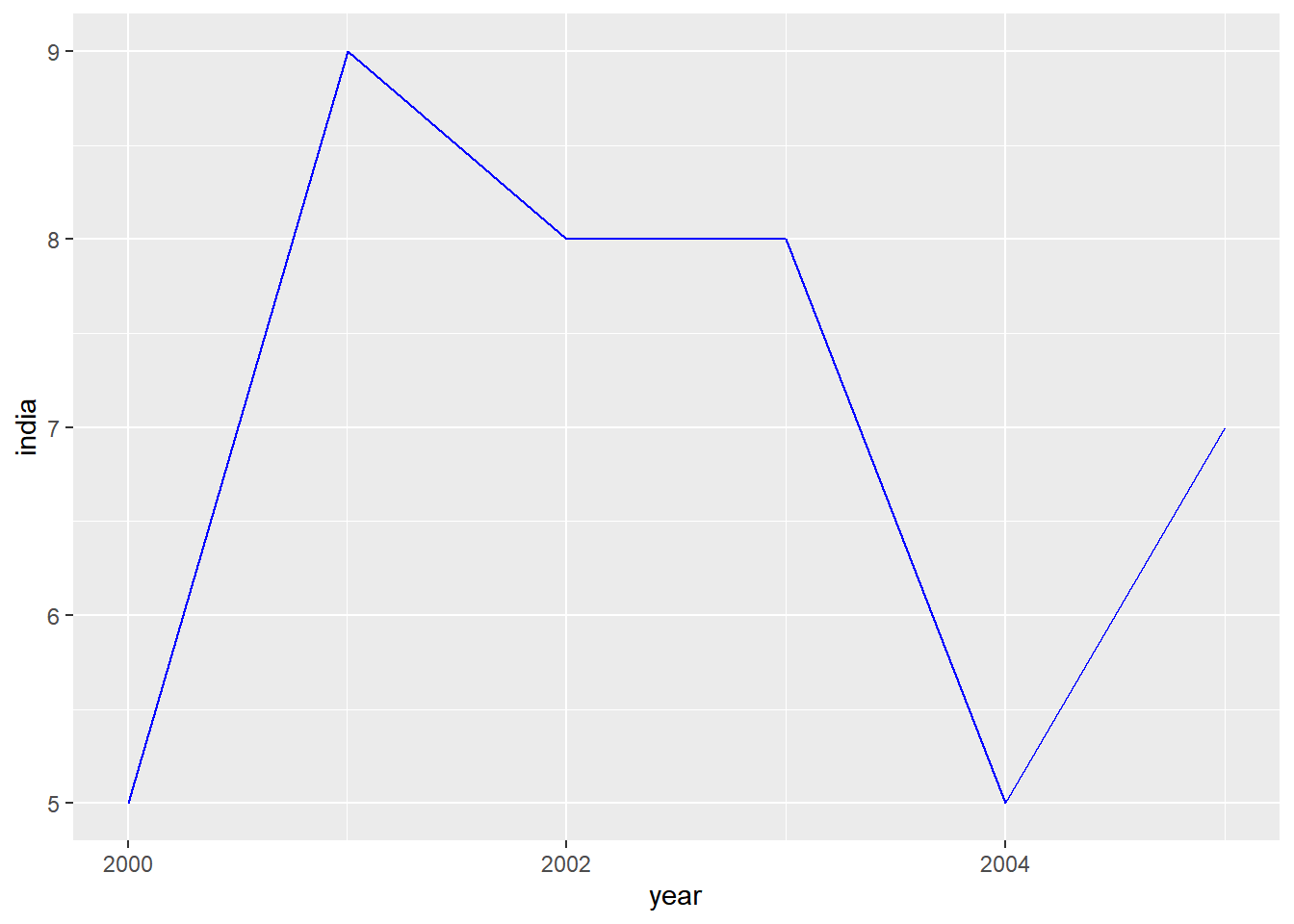#### Line Type

The line type can be modified using the `linetype` argument. It can take 7 different values. You can specify the line type either using numbers or words as shown below:

• 0 : blank
• 1 : solid
• 2 : dashed
• 3 : dotted
• 4 : dotdash
• 5 : longdash
• 6 : twodash

Let us modify the line type to dashed style by supplying the value `2` to the `linetype` argument.

``````ggplot(gdp, aes(year, india)) +
geom_line(linetype = 2)``````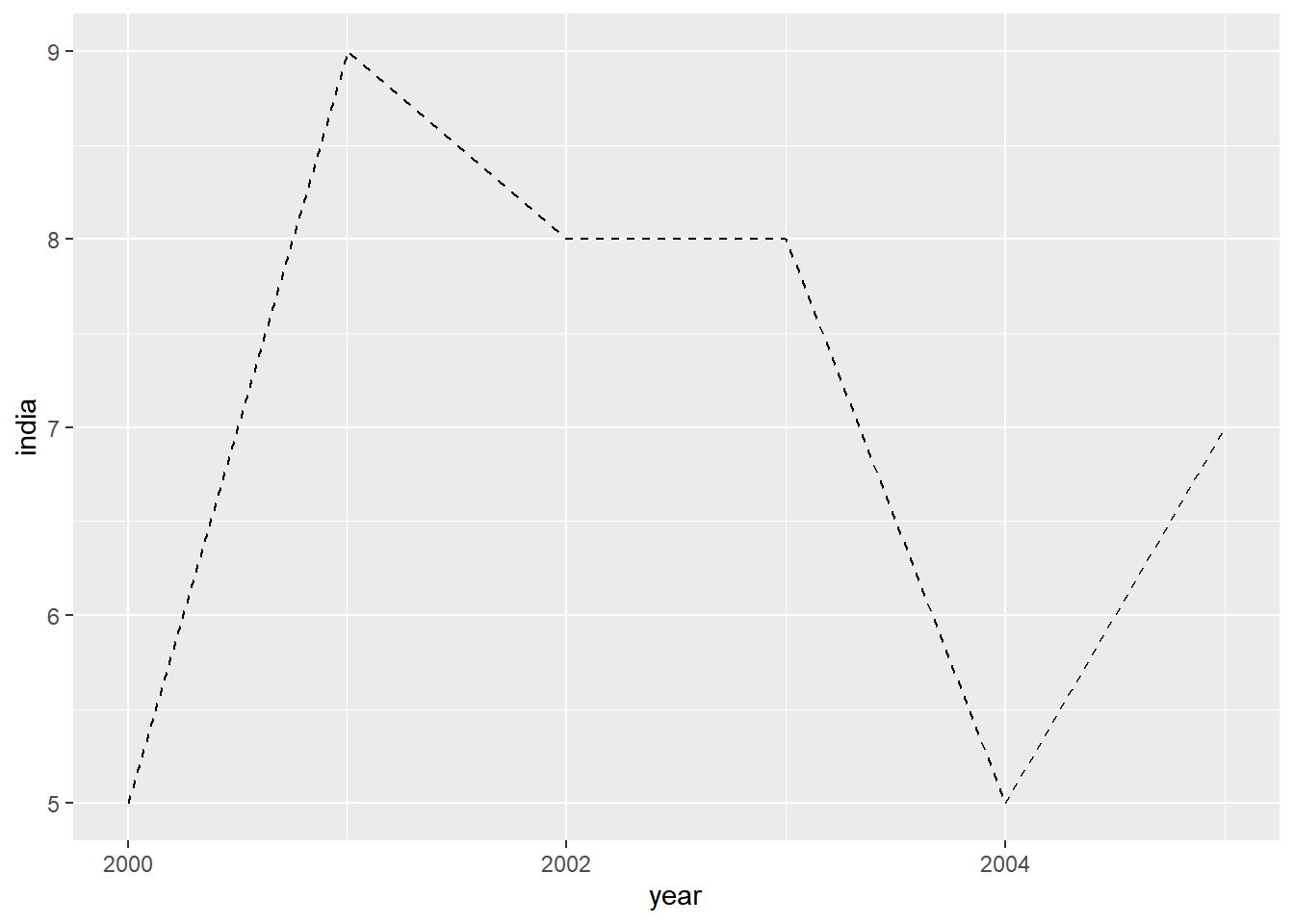The above example can be recreated by supplying the value `'dashed'` instead of `2`.

``````ggplot(gdp, aes(year, india)) +
geom_line(linetype = 'dashed')``````#### Line Size

The width of the line can be modified using the `size` argument. It can take any value above 0 as input.

``````ggplot(gdp, aes(year, india)) +
geom_line(size = 2)``````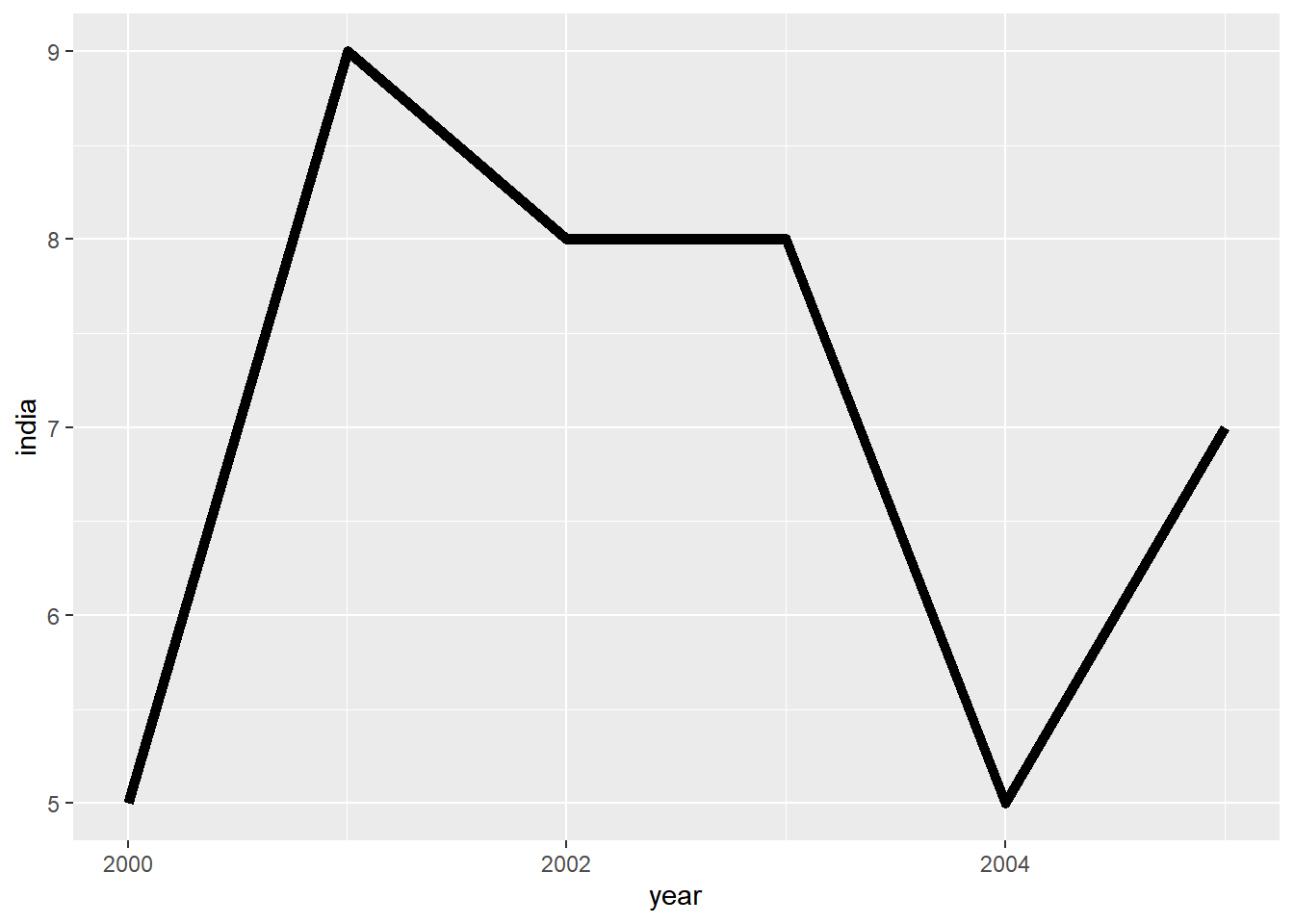#### Modify Data

Now let us map the aesthetics to the variables. The data used in the above example cannot be used as we need a variable with country names. We will use `gather()` function from the `tidyr` package to reshape the data.

``````gdp2 <- gdp %>%
select(year, growth, india, china) %>%
gather(key = country, value = gdp, -year)

gdp2``````
``````## # A tibble: 18 x 3
##    year       country   gdp
##    <date>     <chr>   <dbl>
##  1 2000-01-01 growth      6
##  2 2001-01-01 growth      9
##  3 2002-01-01 growth      8
##  4 2003-01-01 growth      9
##  5 2004-01-01 growth      9
##  6 2005-01-01 growth      8
##  7 2000-01-01 india       5
##  8 2001-01-01 india       9
##  9 2002-01-01 india       8
## 10 2003-01-01 india       8
## 11 2004-01-01 india       5
## 12 2005-01-01 india       7
## 13 2000-01-01 china       8
## 14 2001-01-01 china       5
## 15 2002-01-01 china       6
## 16 2003-01-01 china       8
## 17 2004-01-01 china       9
## 18 2005-01-01 china       8``````

#### Grouped Line Chart

In the original data, to plot GDP trend of multiple countries we will have to use `geom_line()` multiple times. But in the reshaped data, we have the country names as one of the variables and this can be used along with the `group` argument to plot data of multiple countries with a single line of code as shown below. By mapping country to the `group` argument, we have plotted data of all countries.

``````ggplot(gdp2, aes(year, gdp, group = country)) +
geom_line()``````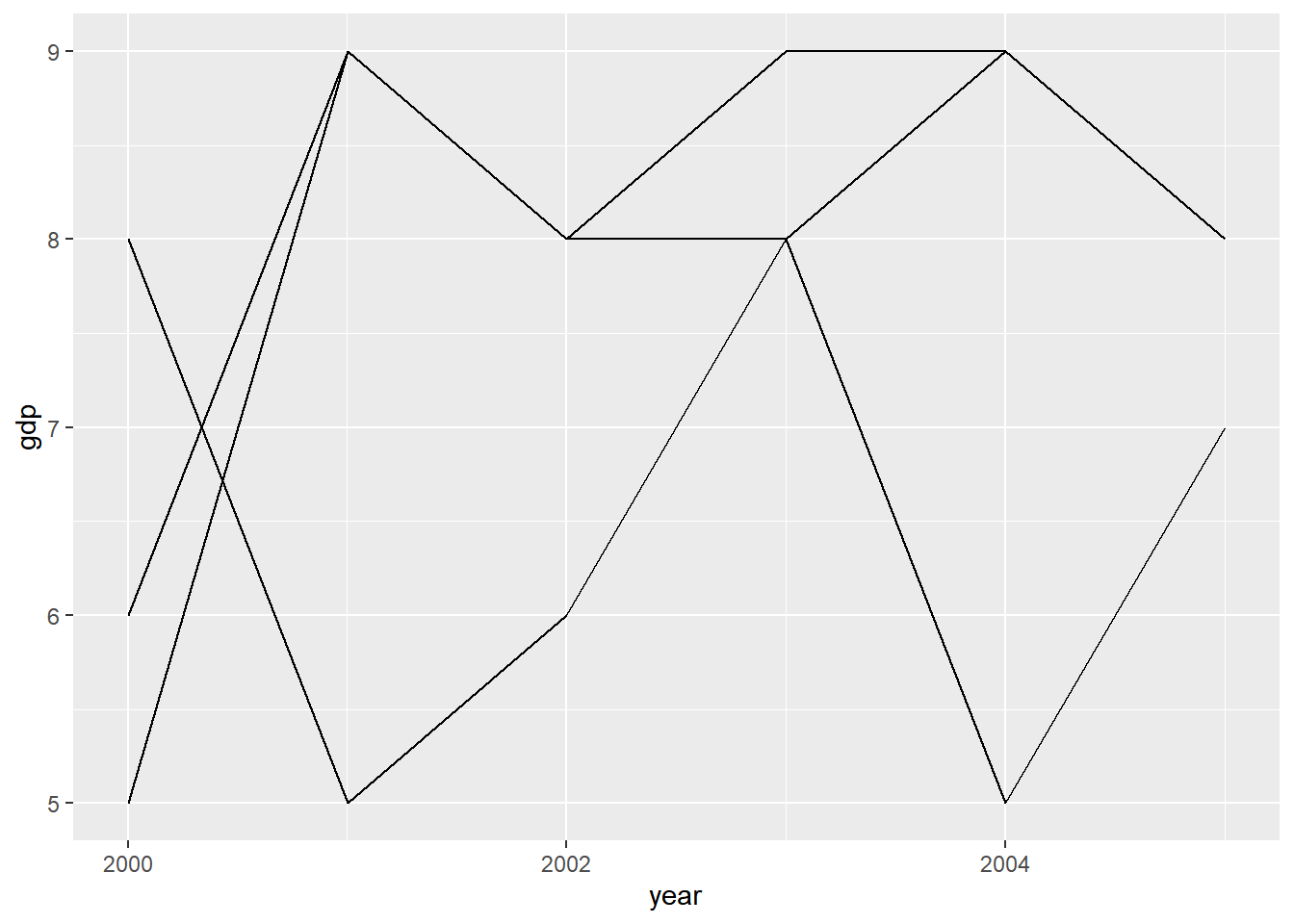In the above plot, we cannot distinguish between the lines and there is no way to identify which line represents which country. To make it easier to identify the trend of different countries, let us map the `color` argument to the variable country as shown below. Now, each country will be represented by line of different color.

``````ggplot(gdp2, aes(year, gdp, group = country)) +
geom_line(aes(color = country))``````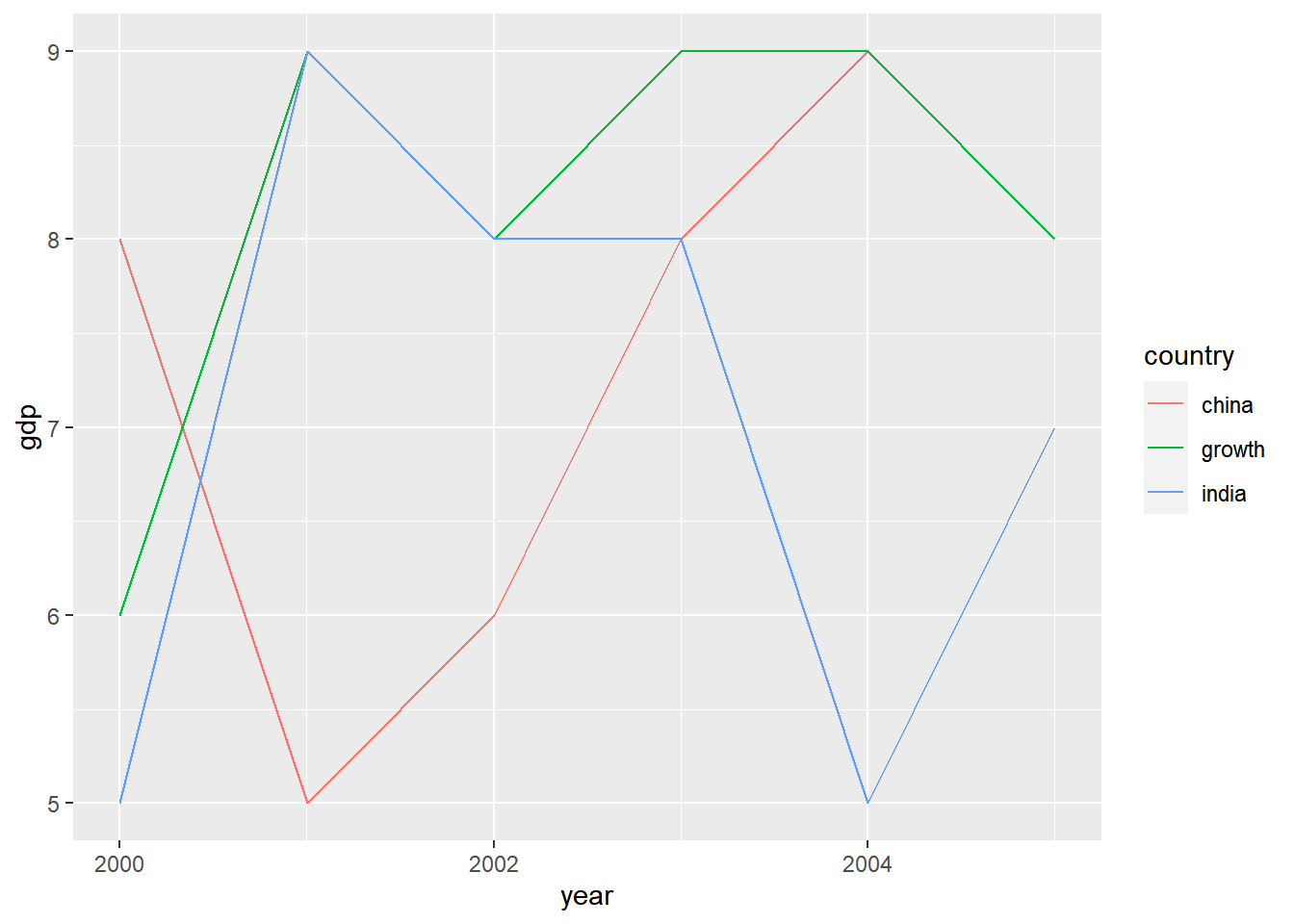We can map `linetype` argument to country as well. In this case, each country will be represented by a different line type.

``````ggplot(gdp2, aes(year, gdp, group = country)) +
geom_line(aes(linetype = country))``````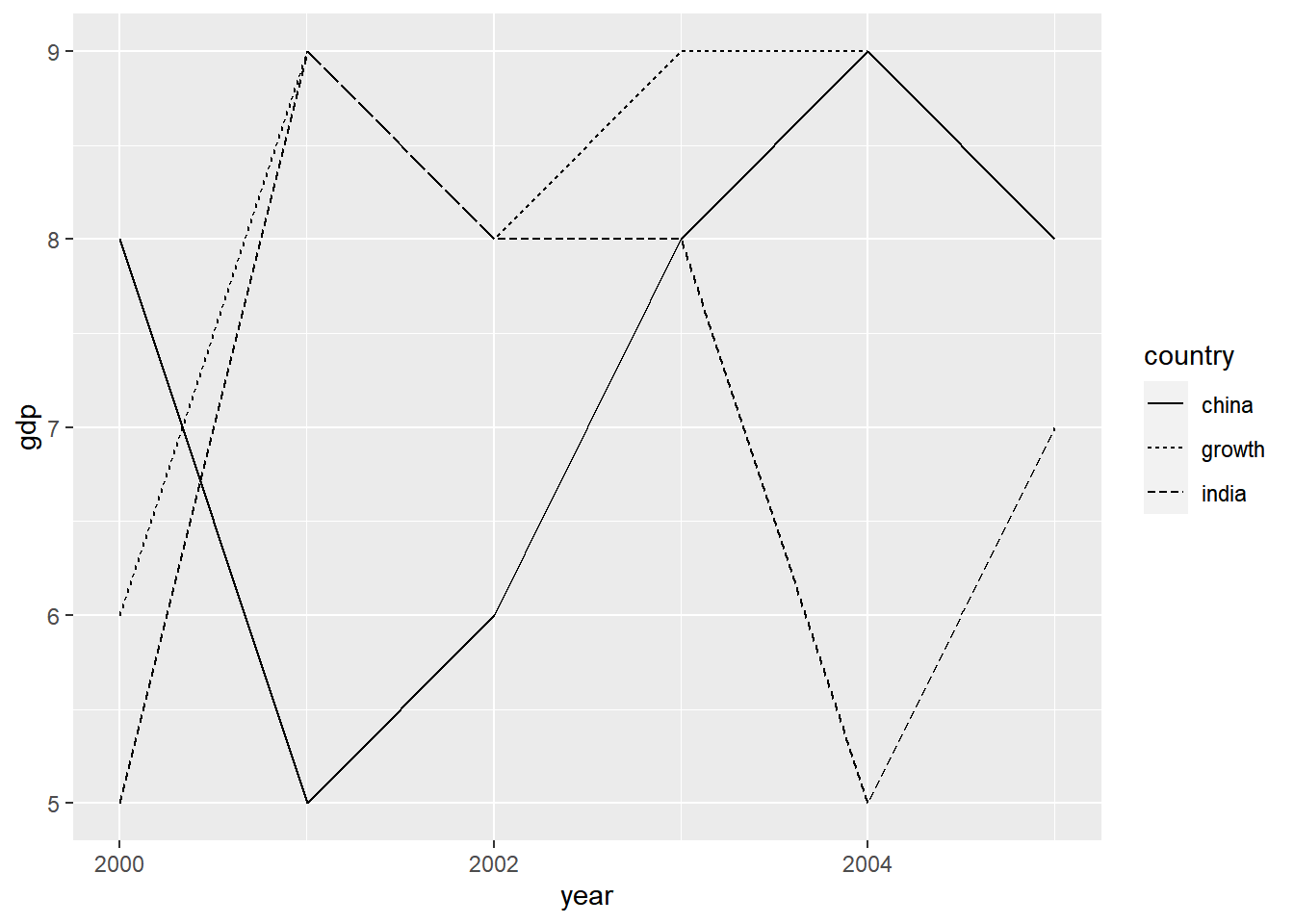We can map the width of the line to the variable country as well. But in this case, the plot does not look either elegant or intuitive.

``````ggplot(gdp2, aes(year, gdp, group = country)) +
geom_line(aes(size = country))``````
``## Warning: Using size for a discrete variable is not advised.``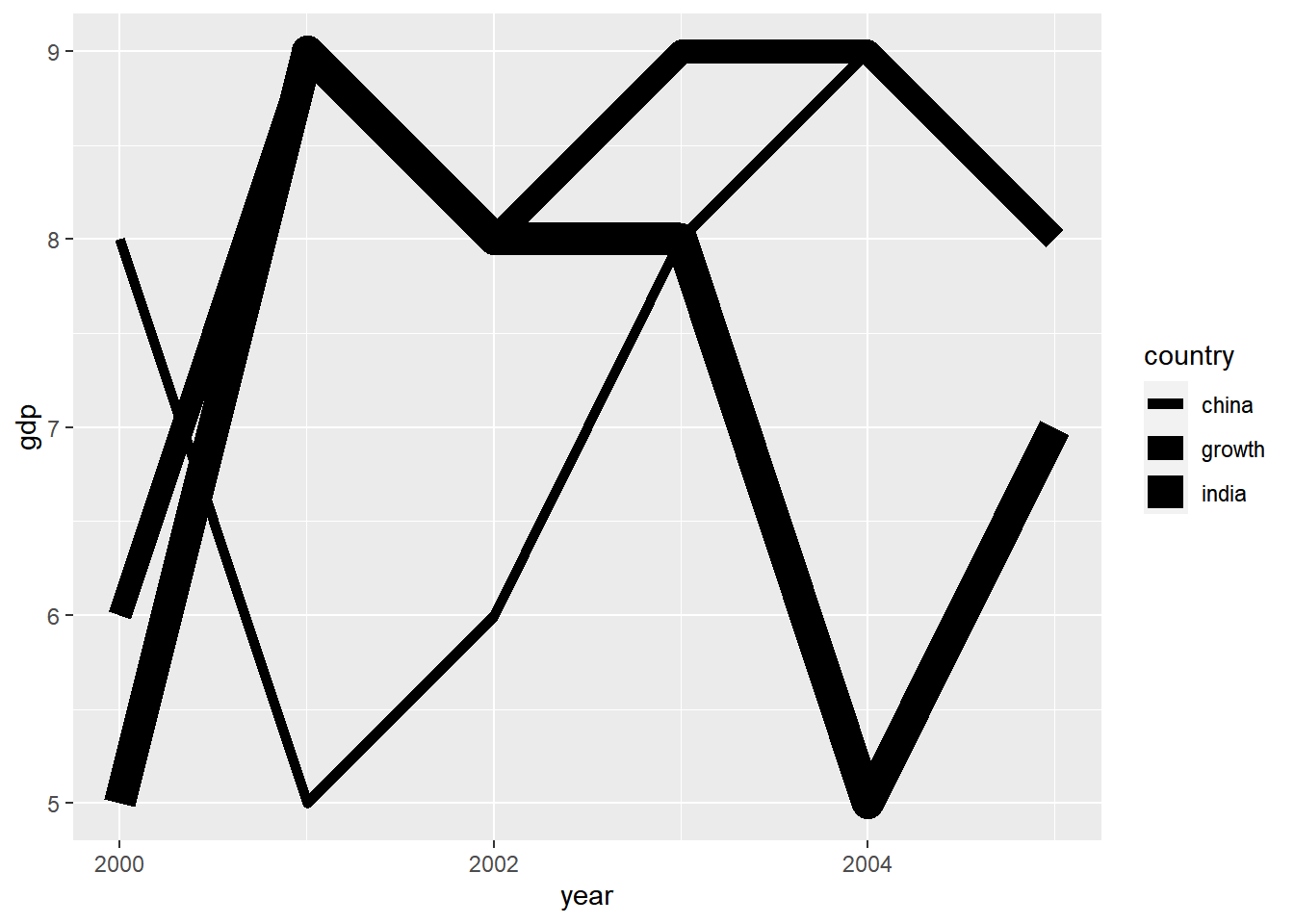Remember that in all the above cases, we mapped the arguments to a variable inside `aes()`.

### Summary

In this post, we learnt to:

• build
• simple line chart
• grouped line chart
• map aesthetics to variables
• modify line
• color
• type
• size

### Up Next..

In the next post, we will learn to build bar plots.